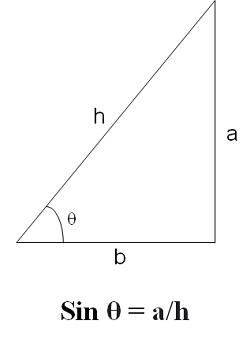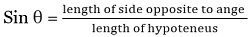# valarray sin() Function in C++ with Examples

C++ valarray sin() Function: Here, we will learn about the sin() function, its usages, syntax and examples.
Submitted by Shivang Yadav, on May 08, 2022

The valarray class in C++ is a special container that is used for holding elements like an array and performing operations on them.

Sin function (mathematical Definition):

Mathematically, sine is a trigonometric function that relate to the angle of a right-angled triangle. Its value is the ratio of the length of side opposite to the angle and the hypotenuses.## std::sin(std::valarray) Function

The sin() function of valarray class is used to find the sin value of each element of the valarray. The method calculates the sine value and return a new valarray consisting of the sine resultant value.

Syntax:

```template< class T >
valarray<T> sin( const valarray<T>& va );

// or
sin(valarrayName)
```

Parameter(s): The method accepts a single parameter. It is the valarray on which the sin() function is applied.

Return Value: The method returns a valarray with an element whose value is equivalent to the sine of the value of the original valarray.

## C++ valarray sin() Function Example 1:

```#include <iostream>
#include <valarray>
using namespace std;

int main()
{
// Declaring valarray
valarray<double> myvalarr = { 1, 0.2, 1.3, 2.4, 5 };

// Printing the elements of valarray
cout << "The elements of valarray are : ";
for (double& ele : myvalarr)
cout << ele << " ";

// Creating a new valarray of sine values
valarray<double> sinValarray;
sinValarray = sin(myvalarr);

cout << "\nThe elements of sin valarray is : ";
for (double& ele : sinValarray)
cout << ele << " ";

return 0;
}
```

Output:

```The elements of valarray are : 1 0.2 1.3 2.4 5
The elements of sin valarray is : 0.841471 0.198669 0.963558 0.675463 -0.958924
```

The angles converted by the sine function are taken in radians and the normal angle we read are in degrees. Here, radian value is equivalent to the angles in degree.

```180° = π radians = 3.14159
90° = π/2 radians = 1.5708
60° = π/3 radians = 1.0472
45° = π/4 radians = 0.785398
30° = π/4 radians = 0.523599
```

Now, let's see the sine values of these.

## C++ valarray sin() Function Example 2:

```#include <iostream>
#include <valarray>
using namespace std;

int main()
{
// Declaring valarray
valarray<double> myvalarr = { 3.14, 1.5708, 1.0472, 0.785398, 0.523599 };

// Printing the elements of valarray
cout << "The elements of valarray are : ";
for (double& ele : myvalarr)
cout << ele << " ";

// Creating a new valarray of sine values
valarray<double> sinValarray;
sinValarray = sin(myvalarr);

// Printing the elements of valarray
cout << "\nThe elements of sin valarray is : ";
for (double& ele : sinValarray)
cout << ele << " ";

return 0;
}
```

Output:

```The elements of valarray are : 3.14 1.5708 1.0472 0.785398 0.523599
The elements of sin valarray is : 0.00159265 1 0.866027 0.707107 0.5
```

Preparation